How Cheenta works to ensure student success?
Explore the Back-Story

# Area of a part of circle | PRMO 2017 | Question 26Try this beautiful problem from the Pre-RMO, 2017, Question 26, based on Area of part of circle.

## Area of part of circle - Problem 26

Let AB and CD be two parallel chords in a circle with radius 6 such that the centre O lies between these chords. Suppose AB=6 and CD=8. Suppose further that the area of the part of the circle lying between the chords AB and CD is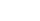where m.n.k are positive integers with gcd(m,n,k)=1. What is the value of m+n+k?

• is 107
• is 75
• is 840
• cannot be determined from the given information

### Key Concepts

Equation

Algebra

Integers

PRMO, 2017, Question 26

Higher Algebra by Hall and Knight

## Try with Hints

A=2[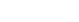]+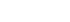+where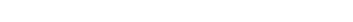or,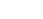or, A=24+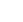or, A=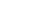(m+n+k)=(48+2+25)=75

## Subscribe to Cheenta at Youtube

Try this beautiful problem from the Pre-RMO, 2017, Question 26, based on Area of part of circle.

## Area of part of circle - Problem 26

Let AB and CD be two parallel chords in a circle with radius 6 such that the centre O lies between these chords. Suppose AB=6 and CD=8. Suppose further that the area of the part of the circle lying between the chords AB and CD iswhere m.n.k are positive integers with gcd(m,n,k)=1. What is the value of m+n+k?

• is 107
• is 75
• is 840
• cannot be determined from the given information

### Key Concepts

Equation

Algebra

Integers

PRMO, 2017, Question 26

Higher Algebra by Hall and Knight

## Try with Hints

A=2[]++whereor,or, A=24+or, A=(m+n+k)=(48+2+25)=75

## Subscribe to Cheenta at Youtube

This site uses Akismet to reduce spam. Learn how your comment data is processed.

### Knowledge Partner